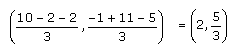# RD Sharma Solutions for Class 10 Maths Chapter 14- Co-ordinate Geometry Exercise 14.4

Applications of section formula comprise the main subject in this exercise. Students will understand how to find the coordinates of the centroid of a triangle as an application of section formula. The RD Sharma Solutions Class 10 can be used as a quick reference for solutions to problems in any chapter of the book. Students can also download the RD Sharma Solutions for Class 10 Maths Chapter 14 Co-ordinate Geometry Exercise 14.4 PDF, which is provided below.

## RD Sharma Solutions for Class 10 Maths Chapter 14 Co-ordinate Geometry Exercise 14.4 Download PDF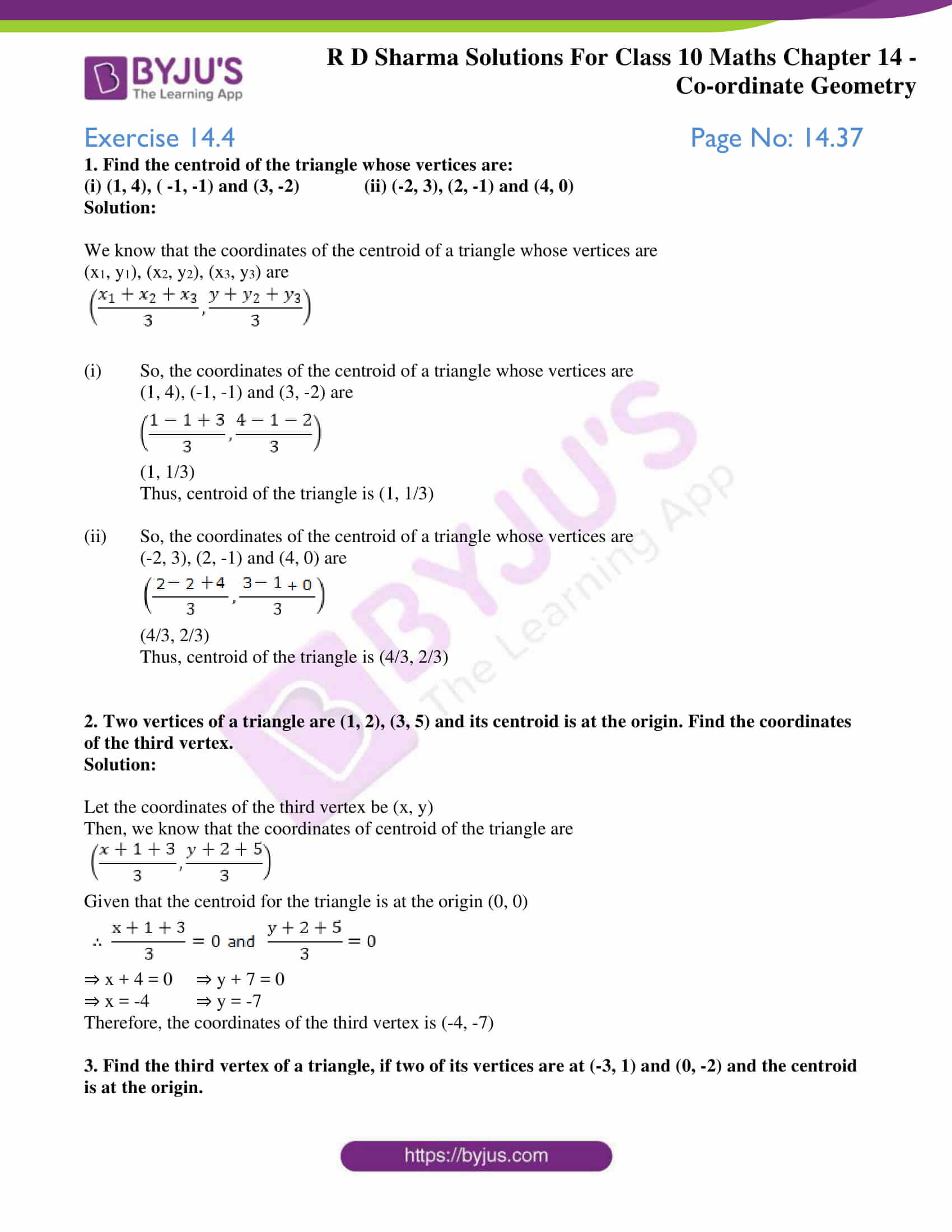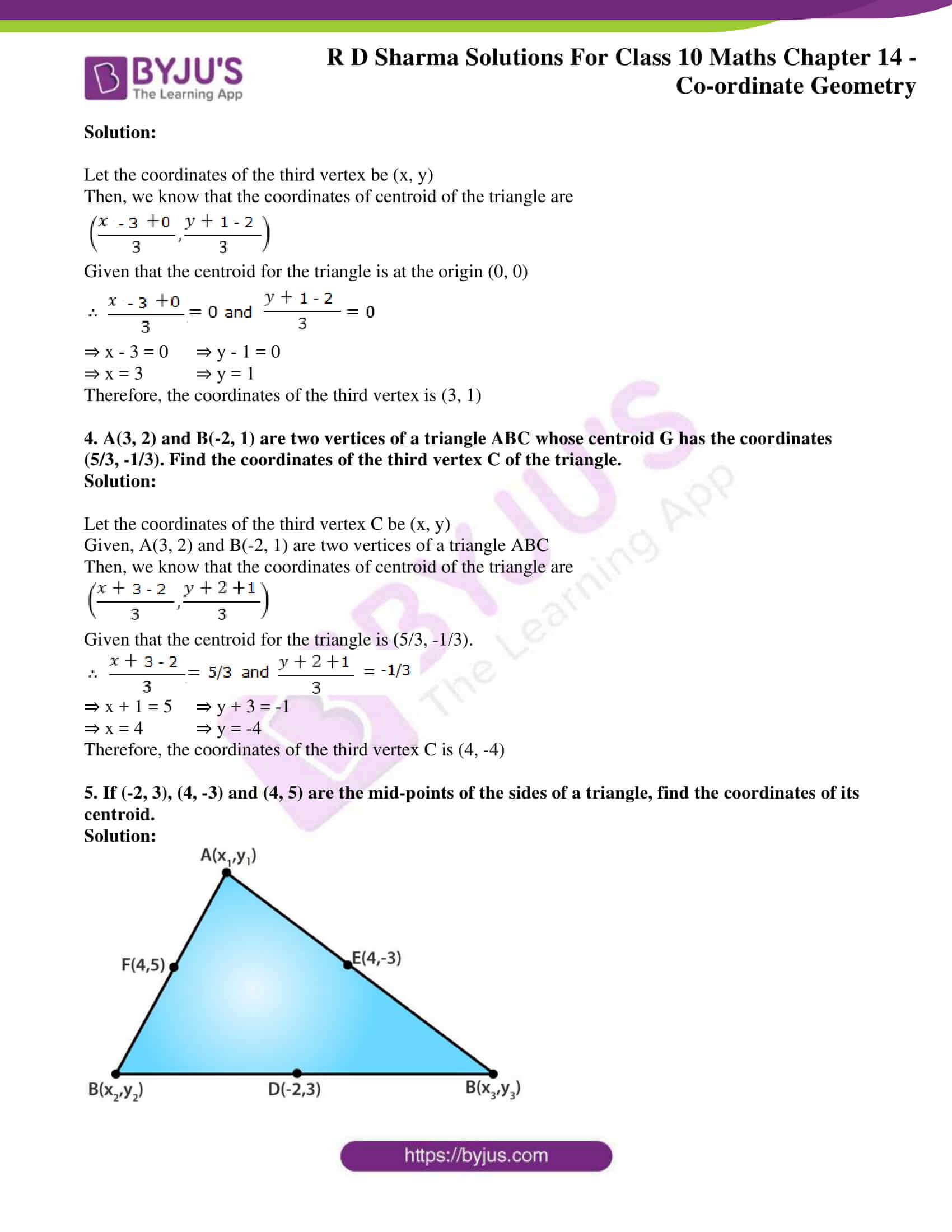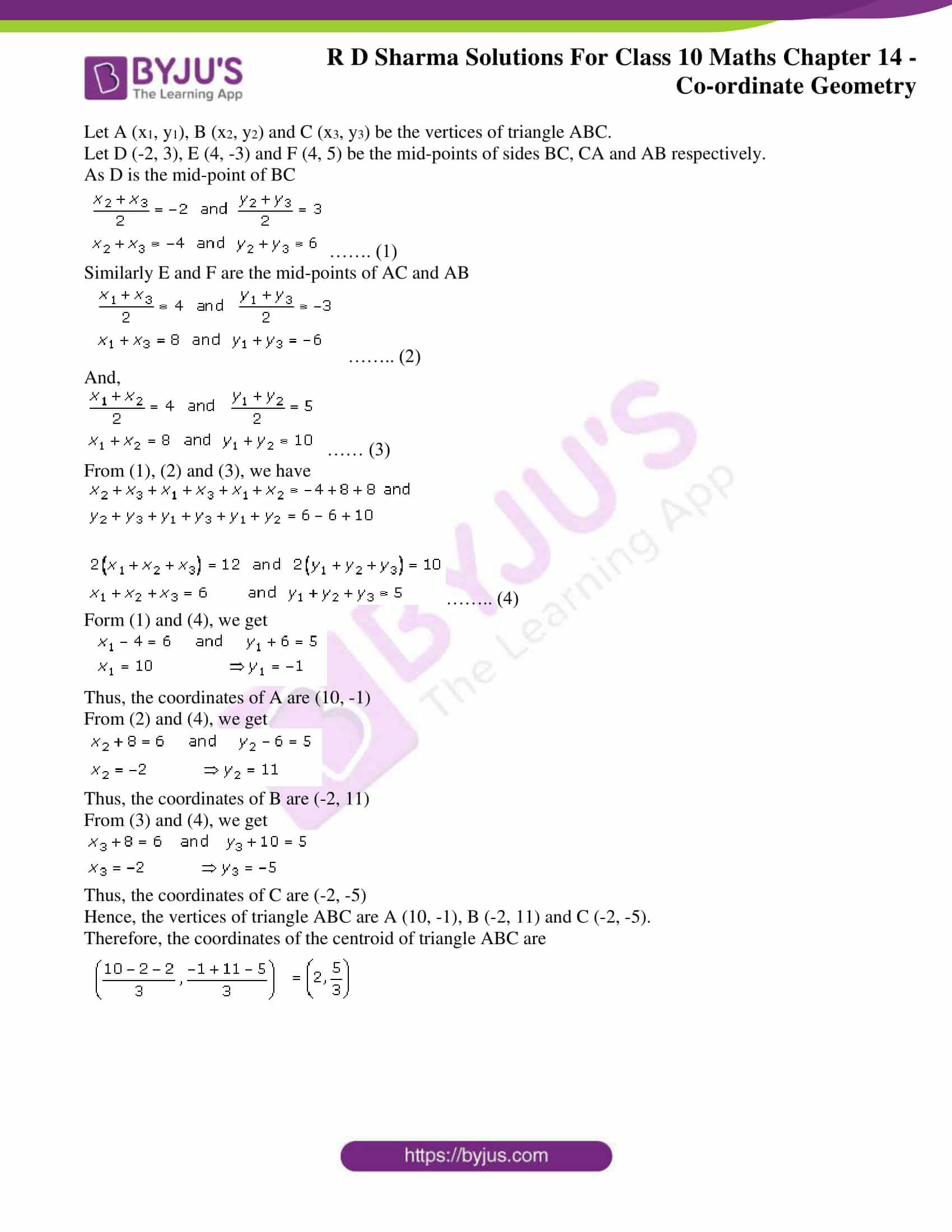### Access RD Sharma Solutions for Class 10 Maths Chapter 14 Co-ordinate Geometry Exercise 14.4

1. Find the centroid of the triangle whose vertices are:

(i) (1, 4), ( -1, -1) and (3, -2) (ii) (-2, 3), (2, -1) and (4, 0)

Solution:

We know that the coordinates of the centroid of a triangle whose vertices are

(x1, y1), (x2, y2), (x3, y3) are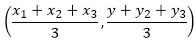(i) So, the coordinates of the centroid of a triangle whose vertices are

(1, 4), (-1, -1) and (3, -2) are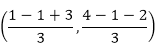(1, 1/3)

Thus, centroid of the triangle is (1, 1/3)

(ii) So, the coordinates of the centroid of a triangle whose vertices are

(-2, 3), (2, -1) and (4, 0) are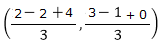(4/3, 2/3)

Thus, centroid of the triangle is (4/3, 2/3)

2. Two vertices of a triangle are (1, 2), (3, 5) and its centroid is at the origin. Find the coordinates of the third vertex.

Solution:

Let the coordinates of the third vertex be (x, y)

Then, we know that the coordinates of centroid of the triangle are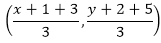Given that the centroid for the triangle is at the origin (0, 0)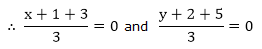⇒ x + 4 = 0 ⇒ y + 7 = 0

⇒ x = -4 ⇒ y = -7

Therefore, the coordinates of the third vertex is (-4, -7)

3. Find the third vertex of a triangle, if two of its vertices are at (-3, 1) and (0, -2) and the centroid is at the origin.

Solution:

Let the coordinates of the third vertex be (x, y)

Then, we know that the coordinates of centroid of the triangle are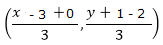Given that the centroid for the triangle is at the origin (0, 0)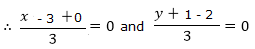⇒ x – 3 = 0 ⇒ y – 1 = 0

⇒ x = 3 ⇒ y = 1

Therefore, the coordinates of the third vertex is (3, 1)

4. A(3, 2) and B(-2, 1) are two vertices of a triangle ABC whose centroid G has the coordinates (5/3, -1/3). Find the coordinates of the third vertex C of the triangle.

Solution:

Let the coordinates of the third vertex C be (x, y)

Given, A(3, 2) and B(-2, 1) are two vertices of a triangle ABC

Then, we know that the coordinates of centroid of the triangle are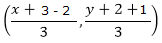Given that the centroid for the triangle is (5/3, -1/3).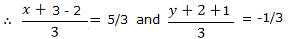⇒ x + 1 = 5 ⇒ y + 3 = -1

⇒ x = 4 ⇒ y = -4

Therefore, the coordinates of the third vertex C is (4, -4)

5. If (-2, 3), (4, -3) and (4, 5) are the mid-points of the sides of a triangle, find the coordinates of its centroid.

Solution: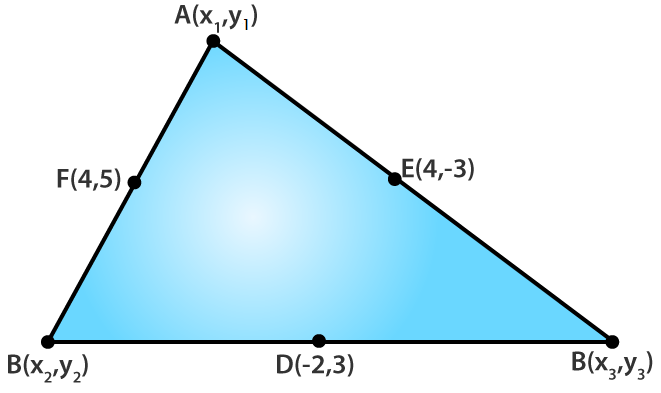Let A (x1, y1), B (x2, y2) and C (x3, y3) be the vertices of triangle ABC.

Let D (-2, 3), E (4, -3) and F (4, 5) be the mid-points of sides BC, CA and AB respectively.

As D is the mid-point of BC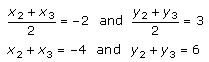……. (1)

Similarly E and F are the mid-points of AC and AB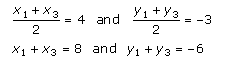…….. (2)

And,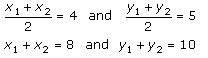…… (3)

From (1), (2) and (3), we have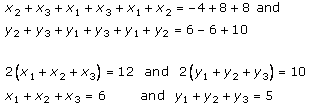…….. (4)

Form (1) and (4), we get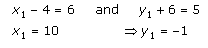Thus, the coordinates of A are (10, -1)

From (2) and (4), we get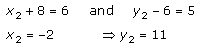Thus, the coordinates of B are (-2, 11)

From (3) and (4), we get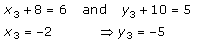Thus, the coordinates of C are (-2, -5)

Hence, the vertices of triangle ABC are A (10, -1), B (-2, 11) and C (-2, -5).

Therefore, the coordinates of the centroid of triangle ABC are